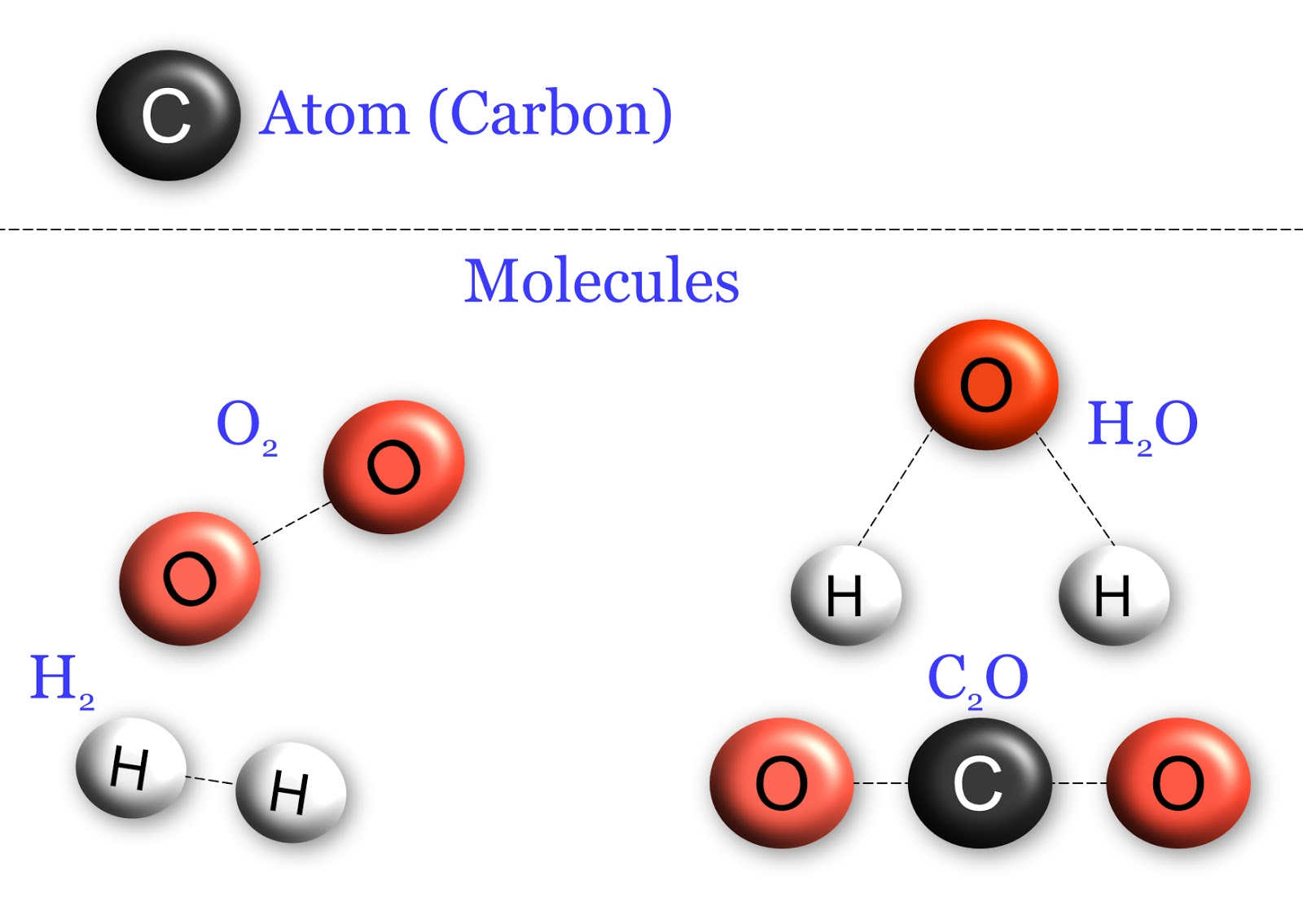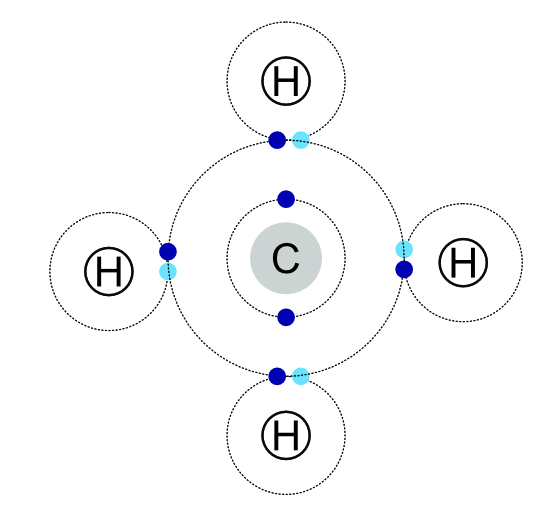Reactions

Chemical Formulae

Chemical formulae are used to represent the structural composition of materials, whether they are atoms, simple molecules or compounds.

1. Atomic: a material made of individual atoms of a single element.
2. Molecules: A material made of atoms joined together by bonds.
3. Compounds: A molecule of more than one type of atom.

Examples:

Atomic: atomic oxygen: O atomic nitrogen: N atomic carbon: C

Molecules: Atmospheric oxygen: O2 Ozone: O3 Carbon dioxide: CO2

Compounds: Glucose: C6H12O6 Carbon dioxide: CO2 Sulfuric acid: H2SO4A subscripted number indicats the number of that atom in the molecule, e.g H2.

A number before a molecular symbol indicates the total number of that molecule; e.g 2H2O

ExampleThis molecule of methane is an example of a compound as it contains carbon and hydrogen bonded together.

There is 1 carbon atom and 4 hydrogen atoms so it has the chemical formula, CH4.Here there are two molecules of methane, this is written as 2CH4.Note when there is only 1 molecule of a chemical the “1” is not written.

Chemical Equations

Chemical equations represent the changes that take place in the arrangement of atoms during a chemical reaction.

The general format is:

Reactant 1 + Reactant 2 → Product 1 + Product 2.

Using the following symbols to represent changes in state or solution.

s: Solid - eg H2O(s) would represent ice.

l: Liquid - eg H2O(l) would represent water

g: Gas - eg H2O(g) would represent steam

↑X(g): Gas given off in an experiment - eg ↑H2(g) hydrogen given off as a product in a reaction.

aq: A chemical dissolved in water to make an aqueous solution - CuSO4(aq), copper sulfate solution.

Due to the law of conservation of mass, the mass of the products used in a reaction must equal the total mass of the products produced by the reaction. The number of each type of atom on each side of the reaction arrow must be the same. This is known as a balanced chemical equation.

Example 1

Calcium + Chlorine → Calcium chloride

Ca(s) + Cl2(g) → CaCl2(s)

Calcium chloride has the formula CaCl2 because Calcium has 2 electrons in its outer orbit, but each chlorine can only accept a single electron as it already has 7 electrons in the outer orbit. Therefore, each calcium must bond with 2 chlorines to lose both spare electrons.

Example 2

Magnesium + Hydrochloric acid → Magnesium chloride + Hydrogen

Mg(s) + 2HCl(l) → MgCl2(s) + ↑H2(g)

Note that there are the same number of each type of atom before and after the reaction.

The equation can be read as: “One atom of magnesium reacts with two molecules of hydrochloric acid to make 1 molecule of magnesium chloride and one molecule of hydrogen gas that is given off in the reaction”.

Half equations

Half equations show the changes that take place to a chemical element during oxidation, reduction reactions or electrolysis. These equations show the number of electrons that are gained or lost by atoms in a reaction.

Example

Sodium + Chlorine → Sodium chloride. 2Na + Cl2 → 2NaCl

Half equations for this reaction

Na → Na+ + e- A sodium atom loses an electron to become a sodium ion.

Cl2 + 2e- → 2Cl- A chlorine molecule gains two electrons to form 2 chlorine ions

Moles

One mole of any chemical contains the same number of atoms/molecules, this number is known as Avogadro’s constant. One mole of a material contains 6.022x 1023 particles.

In practice, if a sample of a material has a mass equal to its relative atomic / molecular mass the sample contains 6.022 x 1023particles or 1 mole of the material.

12g of12C is 1 mole of carbon, containing 6.022x1023 atoms of carbon.

Water has the formula H2O, using the periodic table the relative atomic mass of hydrogen is 1 and oxygen is 16. The relative molecular mass of water is (2xH plus O 2x1 +16) 18.

Therefore, the molar mass of water is 18g.

Sodium chloride has a relative atomic mass of 58.5 (Na = 23, Cl = 35.5). Therefore, 58.5g of sodium chloride is 1 mole of NaCl containing 6.022x1023 molecules.

Mass of individual atoms/molecules

Using Avogadro’s constant and knowing that one mole of a material contains an exact number of particles, the individual mass of an atom or molecule can be calculated.

Mass of atom or molecule = mass of 1 mole ÷ 6.022x 1023

Carbon-12: Mass of12C = 12g / 6.022x 1023 = 1.99x 10-23 g per atom

Sodium chloride: Mass of NaCl = 58.5g / 6.022x 1023 = 9.71x 10-23 g per molecule

Testing Gases

During many chemical reactions, gases are given off. These can be collected and tested to determine which gas has been produced.

Test for Oxygen

The sample of gas is collected in an ignition tube and held upside down to prevent the gas from escaping. A wooden splint is lite away from the tube and the flame is blown out so that the wood has some remaining glowing embers. This is placed into the tube if the tube contains oxygen the splint will relight.

Test for Hydrogen

The sample of gas is collected in an ignition tube and held upside down to prevent the gas escaping, a light splint is held at the opening to the tube. If the tube contains hydrogen the gas will ignite and create a “popping” sound.

Test for Carbon Dioxide

The gas is bubbled through a container of lime water. If the gas is carbon dioxide the lime water will turn milky.

Test for Chlorine

Place a piece of damp blue litmus paper into the container of gas. The paper will turn red then be bleached white. Chlorine gas also has a distinctive greenish colour.

Concentration of Solutions

A solution contains a solute chemical dissolved into a solvent. The more of the solute in the solvent the more concentrated the solution is. Concentration is measured in moles per decimeter, mol/dm^3. A decimeter is equal to 1 litre or 1000 cm^3 of solvent.

Note that 1 ml and 1 cm^3 are equal for pure water, so 1L = 1000ml = 1000cm3 = 1dm^3.

Concentration of a solution (mol/dm3) = moles of solute / volume of solvent (dm^3).

Example 1

1 mol (1M) of salt solution contains 1 mole of NaCl dissolved into 1 dm3 of water. This can be made by dissolving 58.5g of NaCl into 1 litre of water to give a 1 molar solution of salt water (saline).

Example 2

159.5g of copper sulfate is dissolved in 500 cm3 of distilled water, what is the concentration of the resulting solution?

Copper sulfate (CuSO4) molar mass = 159.5g (63.5 + 32 + (4x16)) = 1 M of CuSO4

500 cm^3 of water = 500 / 1000 = 0.5dm^3 of water.

Concentration = moles of solute / volume of solvent

= 1 / 0.5

= 2 M solution of CuSO4

Example 3

How much potassium chloride would have to be dissolved into 250 cm^3 of water to make a 2 M solution?

Potassium chloride: KCl molar mass = (39 + 35.5) = 74.5 g/M

250 cm^3 of water = 250 / 1000 = 0.25 dm^3 of water.

Concentration = moles of solute / volume of solvent.

Therefore: moles of solute = concentration x volume

= 2 x 0.25

= 0.5 M

Mass of KCl requires = Molar mass x mole = 74.5 x 0.5 =37.25 g

Stoichiometry

Stoichiometry uses the law of conservation of mass in reactions to calculate the masses of chemicals taking part in reactions.

The law of conservation of mass tells us that during a reaction no mass is gained or lost. The total mass of the reactants must equal the total mass of the products.

Carbon + Oxygen → Carbon dioxide.

If 12g of carbon are full reacted with the oxygen how much carbon dioxide is produced?

Balanced chemical equation: C + O2 → CO2

This means that 1 M of carbon combines with 1 M of molecular oxygen to give 1 M of carbon dioxide.

1M of C = 12g, 1 M of O2 = 32g (2x16). Total mass of reactants = 12g + 16g = 44g

Therefore 44g of CO2 must also be produced and this is the molar mass of CO2.

The masses of materials used and produced can be used to deduce the balanced equations of a reaction.

10g of sodium are reacted with an excess of chlorine gas to produce 25.39g of sodium chloride, what is the balanced equations for this reaction?

Mass of chlorine used in the reaction 25.39 - 10 = 15.39g (Law of conservation of mass)

Formula for the reactants Na Cl

Atomic / molecular mass 23 35.5

Moles used 10/23 = 0.44 15.39/35.5 = 0.22

Divide by by the smallest value

To find the moles used 0.44 / 0.22 = 2 0.22/0.22 =1

Reactants side of the equation 2Na + Cl2

Balance the equation 2Na + Cl2 → 2NaCl

A reaction will be limited in the amount of products produced by the supply of the reactants. In the above example, the sodium was allowed to react with an excess of chlorine gas to ensure that all the sodium would react. If there had been a limited supply of chlorine, not all the sodium would have reacted and it would be more complicated to work out the masses involved in the reaction.